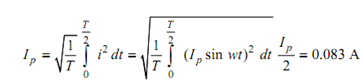## Example Assignment Help

Assignment Help: >> Half-Wave Rectifier - Example

Example:

The input to the half-wave rectifier of given Figure is 120 Vac. If the resistance Rd of the diode during conduction is 20 Ω and the load resistance RL = 1000 Ω , determine the peak, DC and rms load currents and the power dissipated in the load and diode.

Solution

The peak load current is Ip      = Vp /(R L+ Rd  ) = 120(√2 /(1000 + 20)) = 0.166 A . The DC

current is IDC    =Ip/ π = 0.053 A . The rms current, is, hence,The entire input power to the circuit is P1 = PL + Pd. the power dissipated in the load is PL =(Irms)2 RL  = 0.0832  . 1000 = 6.92 W, and the power dissipated in the diode is  Pd=        (Irms  )2Rd = 0.14 W . The total power supplied by the source is, hence, 7.06 W

In the above calculations, we have neglected the 0.7 V voltage drop across the conducting diode. Also note down that the peak reverse voltage across the diode during the non-conducting phase is 120 √2 = 170 V , which the diode should be capable of withstanding.